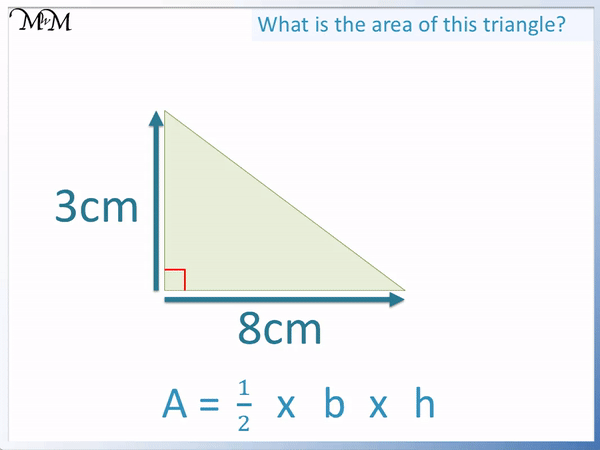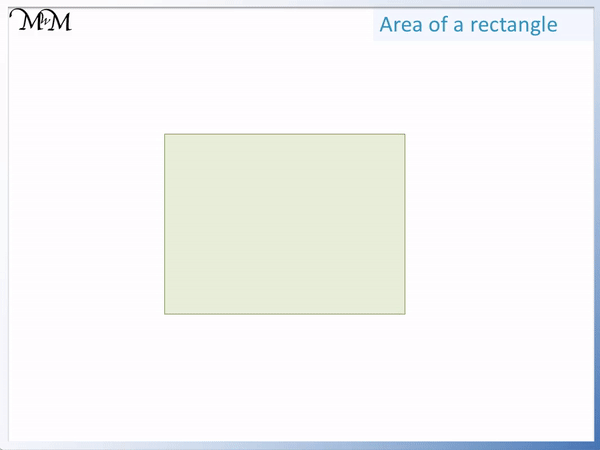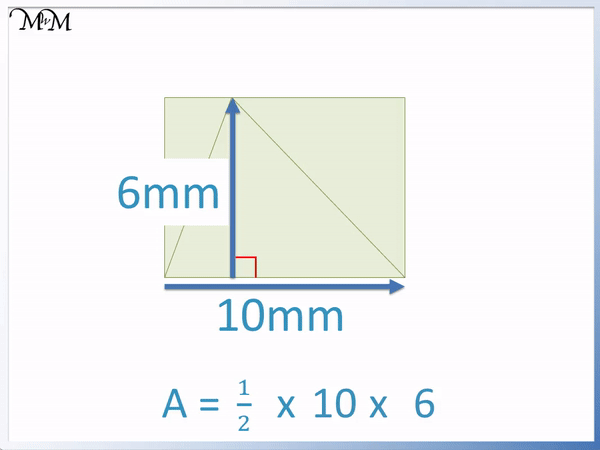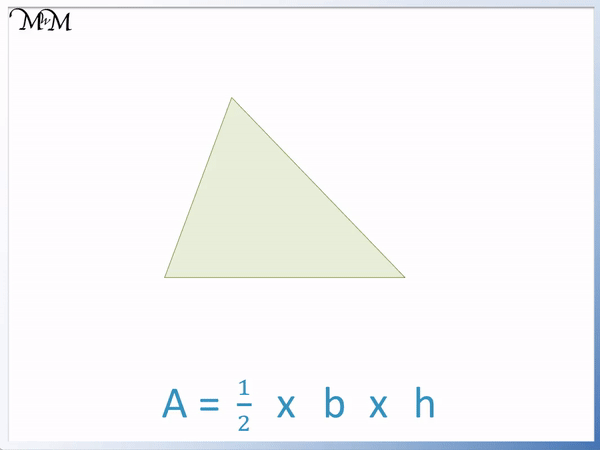# How to Find the Area of a Triangle

How to Find the Area of a Triangle• The area of a triangle is one half times base times height.
• The area formula can be written as   1 / 2   × base × height.
• The base and the height must be at right angles to one another.
• Here the base is 8 cm and the height is 3 cm.
• The area is   1 / 2   × 8 × 3 = 12 cm2.
• The units of area are measured in units squared.
• Since the sides are measured in cm, the area is measured in cm2.
The area of a triangle is   1 / 2   × base × height.

The units of area are measured in units squared.• The area of a triangle is   1 / 2   × base × height.
• The base is 11 m and the height is 8 m.
• We can multiply the base and height and then halve the answer.
• 11 × 8 = 88 and half of this is 44.
• The area of the triangle is 44 m2.
• We could have also halved the 8 first and then multiplied by 11.
• Half of 8 is 4 and then 4 × 11 = 44.
• The units are m2 because the side lengths are measured in metres.Supporting Lessons# Finding the Area of a Triangle

## How to Find the Area of a Triangle

To find the area of a triangle, multiply the base by the height and then divide by 2. Alternatively, divide the base by 2 and then multiply by the height or divide the height by 2 and then multiply by the base.

It does not matter whether you multiply the base and height first and then halve the answer or whether you halve the base or height first and then multiply.

For example, here is a right-angled triangle of base 8 cm and a height of 3 cm.Multiplying the base and height, 8 × 3 = 24. Halve of this answer is 12.

The area is 12 cm2.

The area of a triangle is measured in units squared. Take the unit that the sides are measured in and write this unit squared after your answer. If the sides are measured in cm, then the area units are cm2. If the sides are measured in m, then the area units are m2.

We have multiplied cm by cm in this calculation and so, the area units in this example are cm2.

Instead of multiplying the base by the height first and then dividing by 2, we can halve one of the base or height lengths first.

We will look at the same example and divide by 2 first before multiplying.Since 8 cm is even and 3 cm is not, it is easier to halve the 8 cm length.

Half of 8 is 4 and then 4 × 3 = 12. The area is 12 cm2.

The answer is the the same no matter in what order the numbers are multiplied.

The formula for the area of a triangle is   1 / 2   × base × height. This formula can be more easily written as Area =   1 / 2   bh.

The formula of Area =   1 / 2   bh works for all triangles, no matter what size or shape. As long as the height and base are known, this formula can be used to calculate the area.

Here is an example of using the formula to calculate the area of a triangle.

The base of this triangle is 11 m and the height of the triangle is 8 m.

The value of b = 11 and the value of h = 8.1 / 2   × b × h becomes   1 / 2   × 11 × 8.

We can multiply 11 by 8 first and then halve the answer. 11 × 8 = 88 and then 88 ÷ 2 = 44.

The side lengths are measured in metres and so, the area of this triangle is 44 m2.

When teaching finding the area of a triangle, it is recommended to divide by 2 first before multiplying in order to make the calculation easier.

It is easier to halve the 8 first to get 4 and then multiply this by 11 to get the same answer of 44 m2.

By doing the division first, the multiplication sum uses smaller numbers which are easier to calculate. This method also involves halving a smaller number rather than the larger final answer.

When using the formula to find the area of a triangle, it is important to ensure that the base and height meet at right angles.

Sometimes you may be given all three sides of a triangle. To calculate the area of a triangle, choose two lengths that are at right angles to each other as the base and height. Multiply these lengths and then divide by 2. Ignore any other side lengths.

## Why is the Area of a Triangle One Half Base Times Height

Any triangle can be drawn inside a rectangle that is twice its size. The area of a rectangle is base times height and so, the area of the triangle is one half times base times height.

The area of a rectangle is base × height, alternatively written as length × width.To make a rectangle into a triangle, divide it in half diagonally.Since the area of a rectangle is base × height, the area of a triangle is   1 / 2   × base × height.

Here is another example of a triangle that is half the size of a rectangle.The area of the rectangle is 10 × 6 = 60 mm2 and the area of the triangle is half of this.

The area of the triangle is 30 mm2

Remember that when teaching the area of a triangle, it is easiest to divide either the height or base by 2 first.

Half of 10 is 5 and then 5 × 6 = 30 mm2.Now try our lesson on How to Find the Area of a Parallelogram where we learn how to find the area of a parallelogram.